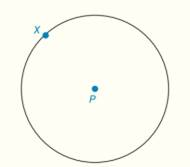Chapter 6.CT, Problem 13CTElementary Geometry For College St...

7th Edition
Alexander + 2 others
ISBN: 9781337614085

Solutions

Chapter
SectionElementary Geometry For College St...

7th Edition
Alexander + 2 others
ISBN: 9781337614085
Textbook Problem

Construct the tangent line to   ⊙ P at point X .To determine

To construct:

The tangent line to P at point X.

Explanation

Given:

P is a circle with point X on it.

Theorem used:

“The line that is perpendicular to the radius of a circle at its endpoint on the circle is a tangent to the circle”.

Approach:

Let us construct a tangent line to P at point X based on the theorem “The line that is perpendicular to the radius of a circle at its endpoint on the circle is a tangent to the circle”.

Construction:

Step 1:

Consider P with point X.

Step 2:

Draw radius PX¯ and extent it to the form PX.

Step 3:

Using X as a center and any length which is less than PX as radius, draw two arcs which intersects the line segment PX at points Y and Z

Still sussing out bartleby?

Check out a sample textbook solution.

See a sample solution

The Solution to Your Study Problems

Bartleby provides explanations to thousands of textbook problems written by our experts, many with advanced degrees!

Get Started

In Exercises 2340, find the indicated limit. 34. limx1x3+12x3+2

Applied Calculus for the Managerial, Life, and Social Sciences: A Brief Approach

Find the mean for the scores in the following frequency distribution table: X f 6 1 5 4 4 2 3 2 2 1

Essentials of Statistics for The Behavioral Sciences (MindTap Course List)

Evaluate the integral. 13. sin5tcos4tdt

Single Variable Calculus: Early Transcendentals

∫(x − cos x)dx = 1 − sin x + C 1 + sin x + C

Study Guide for Stewart's Single Variable Calculus: Early Transcendentals, 8th